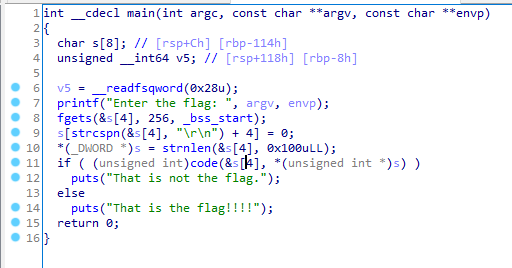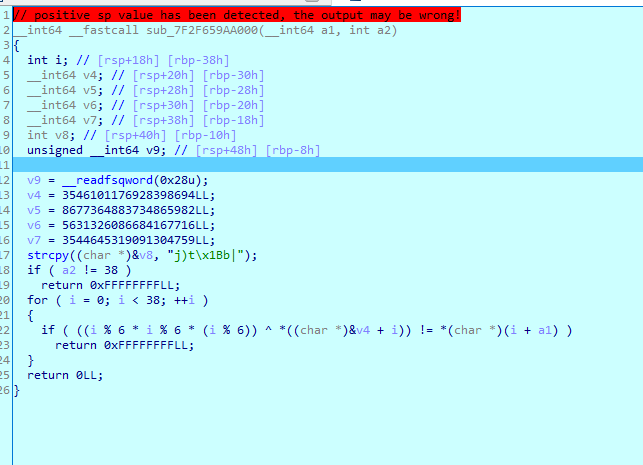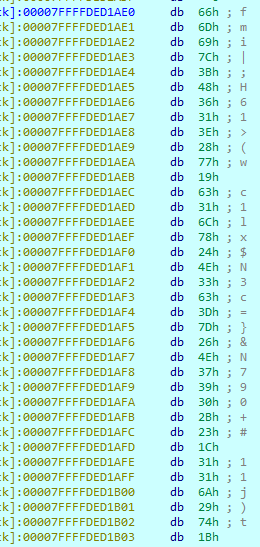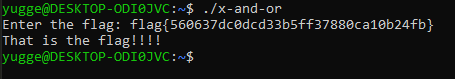# x-and-or

• CTF: 0x41414141 CTF
• Category: reverse
• Writeups

### Given

``Author : M_Alpha``
• ELF file

### Analysis

Running the code promts you with an `Enter the flag:` prompt. when you enter a string it tells you it is the wrong flag.

First we open the code in IDA:This verifies that it would confirm the flag if it is entered. However, the function address seems to be set during runtime. Let's debug the program and step into the function during runtime.We can see that what is sent to this function is a1 (the entered string) and a2 (the length of the string).

On row 18 it checks if the length is 38. The flag must have a length of 38.

Then it steps through my submitted text one char at a time and compare it to:

``((index % 6) * (index % 6) * (index % 6)) ^ MysteryAddr[index]``

Looking at the mystery addr give us this data:That should be all I need to "decode" the flag.

### Implementation

I used the dumped data, implemented that loop I saw in the verifier and converted the result to a string, then printed the string.

``````a = "7C621B74296A31311C232B3039374E267D3D63334E24786C31631977283E3136483B7C696D66"
count = 38
off = 0
out = ""
while (count != 0):
char = int("0x" + a[-(off+2):len(a)-off], 16)
index = 38-count
lol = (index % 6) * (index % 6) * (index % 6)
real = lol ^ char
print(index, chr(real), hex(char), lol)
out += chr(real)
count -= 1
off += 2

print("flag: " + out)``````

Just for the kicks, I sent the outputed flag in the program: